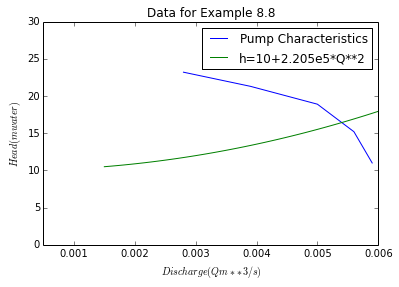# Chapter 8:Pumping of Fluids¶

## Example no:8.1,Page no:320¶

In :
#Variable declaration
dia_cy=110e-3  #Cylinder diameter
stro=230e-3  #stroke
l_su=6  #Suction line length
d_su=50e-3  #Suction line diameter
lvl_wtr=3  #level of the water in the suction tank
atm_P=10.36

#Calculation
import sympy
import math
N=sympy.Symbol('N')    #Maximum speed
#at
t=0                    #Initially
max_acc=0.5*stro*(2*math.pi*N)**2*math.cos(2*math.pi*N*t)  #Maximum acceleration
max_2=(dia_cy/d_su)**2*max_acc   #Maximum acceleration  of liquid in suction pipe
acc_F=max_2*(math.pi/4.0)*d_su**2*l_su*1000   #Accelerating force on the liquid[ N]
P=max_2*l_su*1000     #Pressure drop in suction line N/m**2
P=P/(1000*9.81)     #in m water

#Result
print"Maximum speed at which the pump can run =",round(n,3),"Hz"

Maximum speed at which the pump can run = 0.677 Hz


## Example no:8.2,Page no:343¶

In :
#Variable declaration
rho_l=800  #Density of liquid
Meu_l=0.5e-3 #Viscosity of liquid
Q=0.0004 #Volumetric flow rate
liq_depth=0.07
d=25e-3 #Diameter of pipe used
p_v_r=1e3 #Pressure of vapor in reboiler

#Calculation
import math
A=math.pi/4*d**2 #Cross sectional area of pipe
u=Q/A #Velocity in pipe
Re=d*u*rho_l/Meu_l #Reynolds no.
phi=0.0028
hf_l=(4*phi*u**2)/(d*9.81)
l=10
hf=hf_l*l
ho=Z+hf

#Result
print"The minimum height required =",round(ho,1),"m"

The minimum height required = 2.3 m


## Example no:8.3,Page no:356¶

In :
#Variable declaration
Q=0.1 #Flow rate of air suppplied by compressor
T=273 #Temperature
P=101.3e3 #Pressure
P2=380e3 #Air compressed to a pressure
T2=289 #Suction Temperature
l=0.25 #Length of the stroke
u=4 #Speed
c=4/100.0 #Cylinder clearance
Gamma=1.4

#Calculation
import math
V=Q*T2/(u*T) #Volume per stroke
R=P2/P #Compression ratio
Vs=V/(1+c-(c*(R)**(1/Gamma)))
A=Vs/l #Cross sectional Area of cylinder
d=(A/math.pi*4)**0.5 #Diameter of cylinder
W=P*V*(Gamma/(Gamma-1))*((R)**((Gamma-1)/Gamma)-1)

#Result
print"\n Diameter of cylinder =",round(d,2),"m"
print"\n Theoretical power requirements =",round(W*4/1e3,1),"kW"

 Diameter of cylinder = 0.38 m

Theoretical power requirements = 17.2 kW


## Example no:8.4,Page no:357¶

In :
#Variable declaration
T=290 #Temperature at which compression takes place
P1=101.3e3 #Initial pressure
P2=2065e3 #Final pressure
eta=0.85 #Mechanical efficiecy
c1=4/100.0 #Clearance in cylinder 1
c2=5/100.0 #Clearance in cylinder 1

#calculation
import math
R=P2/P1                         #Overall compression ratio
V_spe=22.4/28.8*T/273.0 #Specific volume of air at 290 K
W=P1*V_spe*2*(1.25/(1.25-1))*(R**.1-1)
W_act=W/0.85
W_it=P1*V_spe*math.log(R)
eta_it=100*W_it/W_act
Gamma=1.4
W_ie=P1*V_spe*(Gamma/(Gamma-1))*((R)**((Gamma-1)/Gamma)-1)
eta_ie=100*W_ie/W_act
Vs1=V_spe/(1+c1-(c1*(R)**(1/(2*2.5))))
Vs2=V_spe*(1/R)**0.5/(1+c2-(c2*(R)**(1/(2*2.5))))
ratio=Vs1/Vs2

#Result
print"(a).Energy supplied to the compressor, that is the work of compression =",round(W_act*1e-3,1),"kJ/kg"
print"(b).Isothermal efficiency =",round(eta_it),"%"
print"(c).Isentropic efficiency =",round(eta_ie),"%"
print"(d).The ratio of the swept volumes in the two cylinders =",round(ratio,2)

(a).Energy supplied to the compressor, that is the work of compression = 346.5 kJ/kg
(b).Isothermal efficiency = 73.0 %
(c).Isentropic efficiency = 116.0 %
(d).The ratio of the swept volumes in the two cylinders = 4.48


## Example no:8.5,Page no:360¶

In :
#Variable declaration
Q_l=7.5e-4
rho_l=1200.0
h=20.0
P=450e3
eta=30.0/100.0
P_atm=101.3e3
Gamma=1.4
G=Q_l*rho_l #Mass flow of liquid
W=G*9.81*h
W_act=W/eta
M=28.9
va=22.4/M

#Calculation
import sympy
import math
x=sympy.Symbol('x')
Ga=sympy.solve(P_atm*va*x*math.log(P/P_atm)-W_act)
Q=Ga*va
Power=(P_atm*Q)*(Gamma/(Gamma-1))*((P/P_atm)**((Gamma-1)/Gamma)-1)
Power_reqd=Power/1000

#Result
print"Power requirement of the pump =",round(Power_reqd,3),"kW"

Power requirement of the pump = 0.734 kW


## Example no:8.6,Page no:360¶

In :
#Variable declaration
P1=101.3e3
Q_watr=0.01
depth=100
d=100e-3
depth_watr=40
Q_air=0.1
P2=800e3
Gamma=1.4
#V1=Q_air

#Calculation
G_watr=Q_watr*1000 #Mass flow of water
W=G_watr*depth_watr*9.81
E=P1*Q_air*(1.4/0.4)*((P2/P1)**(0.4/1.4)-1) #     equation 8.37
effi=W/E*100
P=345e3
v1=8314*273/(29*P)
v2=8314*273/(29*P1)
G_air=Q_air/v2  #mass flowrate of the air is:
Q_mean=G_air*v1 #Mean volumetric flowrate of air
Q_tot=Q_watr+Q_mean #Total volumetric flowrate
A=math.pi/4*d**2 #Area of pipe
v_mean=Q_tot/A

#Result
print"Efficiency =",round(effi,1),"%"
print"Mean velocity of the mixture =",round(v_mean,2),"m/s"

Efficiency = 13.8 %
Mean velocity of the mixture = 5.01 m/s


## Example no:8.7,Page no:369¶

In :
#Variable declaration
d=40e-3 #Internal Diameter of the pipe
l_p=150.0  #Lendth of pipe
Q_watr=600e-6 #Flow of water
h1=10.0  #Vertical Height
h2=2.0  #head lost across heat exchanger
eta=60.0/100.0  #Efficiency of pump

#Calculation
import math
A=math.pi/4*d**2  #Area for flow
u=Q_watr/A  #Velocity
#At 320 K,
Meu=0.65e-3
rho=1000
Re=d*u*rho/Meu
phi=0.004  #for a relative roughness of 0.005
l=l_p+h1+(260*d)
hf=4*phi*l*u**2/(d*9.81)
h_tot=hf+h1+h2 #Total head to be developed
G=Q_watr*rho # Mass flow of water
P_r=G*h_tot*9.81  #Power Required
P_s=P_r/eta  #Power Supplied

#Result
print"Power Required =",round(P_s,2),"W"

Power Required = 133.26 W


## Example no:8.8,Page no:370¶

In :
#Variable declaration
%matplotlib inline
eta=0.50
Q=[0.0028,0.0039,0.0050,0.0056,0.0059]
h=[23.2,21.3,18.9,15.2,11.0]
Q1=linspace(0.0015,0.0060,42)
h1=10+2.205e5*Q1**2
plot(Q,h,label="Pump Characteristics")
plot(Q1,h1,label="h=10+2.205e5*Q**2")
plt.ylim(0,30)
plt.xlim(0.0005,0.006)
title("Data for Example 8.8")
plt.xlabel("$Discharge (Q m**3/s)$")
plt.ylabel("$Head (m water)$")
legend()
#showing the intersection point
x1=[0,0.0054]
y1=[16.43,16.43]
x2=[0.0054,0.0054]
y2=[0,16.43]
#plot(x1,y1,x2,y2)
Q_r=0.0054
h_r=10+2.205e5*Q_r**2
P=Q_r*1000*h_r*9.81/eta

#Result
show()
print"\n The discharge at the point of intersection between\n the purnp characteristic equation =",Q_r,"m**3/s"
print"\n Power required=",round(P*1e-3,2),"kW"

Populating the interactive namespace from numpy and matplotlibThe discharge at the point of intersection between
the purnp characteristic equation = 0.0054 m**3/s

Power required= 1.74 kW


## Example no:8.10,Page no:375¶

In :
#Variable declaration
Meu_H2=0.009e-3  #Viscosity of hydrogen
P2=2e6  #Downstream Pressure
P1=2.5e6 #Upstream pressure
P_m=(P1+P2)/2 #Mean Pressure
T=295.0 #Temperature of the gas
l=500.0 #Length of the pipe used
d=50e-3 #diameter of pipe used

#Calculation
rho_H2=2*P_m*273.0/(22.4*101.3e3*T) #Density of hydrogen at the mean pressure
A=math.pi*d**2/4.0 #Area of the pipe
eta=0.60  #Efficiency of the pump
v_m=1/rho_H2
phi=0.0024
x=sympy.Symbol('x')
G=sympy.solve((x/A)**2*math.log(P1/P2)+(P2-P1)*rho_H2+4*phi*l/d*(x/A)**2)
P=round(G,1)*P_m*v_m*math.log(P1/P2)/eta

#Result
print"Mass flow rate =",round(G,1),"kg/s"
print"Power required =",round(P*1e-3,2),"kW(approx)"

Mass flow rate = 0.2 kg/s
Power required = 91.19 kW(approx)